# Analysis and Optimization of the Performance of Hydrogenated Amorphous Silicon Solar Cell

Analysis and Optimization of the Performance of Hydrogenated Amorphous Silicon Solar Cell
Laboratory of Semiconductor Devices Physic, University of Tahri Mohamed, PB 417, Bechar (08000), Algeria

Laboratoire of Semiconductor and metallic materials, University of Mohammed Khider,B.P. 145, Biskra 07000, Algéria

Corresponding Author Email:
ayat_leila@yahoo.fr
Page:
151-158
|
DOI:
https://doi.org/10.14447/jnmes.v24i3.a02
3 February 2021
|
Accepted:
5 July 2021
|
Published:
30 September 2021
| Citation

OPEN ACCESS

Abstract:

As the name implies, hydrogenated amorphous silicon (a-Si: H) is composed of silicon atoms which are in a disordered configuration away from all Bravais lattices. The hydrogenated amorphous silicon was manufactured in 1969 where there was a renewed interest in non-hydrogenated amorphous silicon. The use of hydrogenated amorphous silicon as the active material in solar cells inefficient but cheap, is currently much studied. We have presented in this work, the results of the numerical simulation of a-Si: H solar cell by the wxAMPS (Analysis of Microelectronic and Photonic Structures) software and the results were compared with those found experimentally, we find a good agreement. For the efficiency there was a difference of 0.17%. We also study the influence of the thickness of a-Si: H intrinsic layer on the photovoltaic parameters of the solar cell. This allows considering the use of amorphous thinner layers for photovoltaic applications. The efficiency has a maximum value of 7.117 %, corresponding to a intrinsic layer thickness of 560 nm. The using a-Si:H alloys provide a good solution to enhance a-Si:H solar cell performance. The use of a-SiC:H as a p-type window layer in amorphous silicon solar cells is one of its primary photovoltaic applications. The wide band gap of p-a-SiC:H alloy used as window layer for minimizing the optical loss. To improve the efficiency of a-Si:H solar cell, we have study by simulation the performance of the (p) a-SiC:H/(i) a-Si:H/ (n) a-Si:H heterojunction solar cell. The effects of a-SiC:H window layer on the photovoltaic performance have been investigated, where the best initial conversion efficiency of 10.59%.

Keywords:

a-Si:H, alloys, solar cell, numerical simulation

1. Introduction

Solar cells convert the energy are photons coming from the Sun into electricity the voltaic effects the photovoltaic affect. The photovoltaic effects are the generation electron-hole pairs in a material under light exposure, and their separation by an internal Electric field. Solar cells were first commercially introduced in the late 1950's in space application for powering satellites. However, terrestrial applications did not take long to be developed, and nowadays there is a broad choice of promising solar cell technologies for satisfying the energy demand in a sustainable and environmentally friendly way. Amorphous silicon thin-film cells are the oldest and most mature type of thin-film. They are made of non-crystalline silicon, unlike typical solar-cell wafers. Amorphous silicon is cheaper to manufacture than crystalline silicon and most other semiconducting materials.

Amorphous silicon is also popular because it is abundant, nontoxic, and relatively inexpensive. However, the average efficiency is very low, 10 percent . Hydrogenated amorphous silicon is a disordered semiconductor whose optoelectronic properties are governed by the large number of defects present in its atomic structure. Silicon atoms in amorphous silicon largely retains the same basic structure as that of crystal silicon: each silicon atom is connected by covalent bonds to four other silicon atoms arranged as a tetrahedron.

Amorphous silicon is a thin-film material without long range atomic order that can be deposited by a variety of techniques suitable for large area production. In this form, however, amorphous silicon has a high density (of the order of 1019cm-3) of coordination defects (“dangling bonds”) corresponding to departures from the local tetrahedral coordination between four silicon atoms. They act as recombination canters to greatly reduce the carrier lifetime and the carrier diffusion and drift lengths, and to pin the Fermi energy in such a way that the material cannot be effectively doped n- or p type and is not electronically useful. It was discovered, however, that the incorporation of about 10% hydrogen (from glow- discharge decomposition of silane gas) during the deposition process greatly reduces the density of these defects to about 10l6 cm-3 , and this hydrogenated material can then be doped n- or p type to make useful semiconductor devices . A promising new thin-film silicon alloy, a-Si:H, had been discovered .

The present report is devised into two sections: the first section describes the simulation model and define the materials that are used to build the structure as well as the contacts. The results obtained by wxAMPS-1D simulator are validated by comparing with those found experimentally. We will also study by simulation, the influence of the thickness of the intrinsic layer in a-Si: H on the photovoltaic parameters (Vco, Jsc, FF, η) of the solar cell.

Several possibilities exist to improve the conversion efficiency of a solar cell. On the one hand, the interfaces between the window materials, the electrodes and the intrinsic zone (active zone) must be of good quality. On the other hand, a p-type wide bandgap semiconductor material can be used as a window layer to improve light transmission. This same layer must be of high conductivity to increase the electrical potential in the structure and reduce the effects of series resistance. This leads to a better concordance between the different layers of the structure.

The P-a-Si1-xCx:H alloy is popularly known as a wide band gap semiconducting alloy. It was demonstrated in the 1980s that application of the P-a-Si1-xCx:H alloy leads to improved performance of a solar cell with better blue response of its quantum efficiency (QE). There are few other well-known wide band gap alloy materials available, however one interesting advantage of the P-a-Si1-xCx:H is that both the C and Si are four- fold coordinated atoms, and hence a suitably prepared material may attain wider optical gap with good stability .

In section tow, we have studied the effect of the a-sic: h as window layer on the performance of the photovoltaic cell based on a-si: h. it has been found that the best structure must have a thin window layer, and a doping higher than 1019 cm-3. we have achieved the considerable initial conversion efficiency of 10.59%.

2. Theory

The hydrogenated amorphous silicon (a-Si:H) pin solar cell has been extensively investigated since its introduction by Carlson and Wronski . These extensive investigations are mainly due its continuous advancement in photovoltaic research and development. Amorphous silicon (a-Si:H) alloys have now become an attractive avenue in thin film technology due mainly to its material availability; ease of fabrication and for large scale production capability . The disordered nature of the a-Si:H material gives it greater flexibility in tailoring improved photovoltaic properties and solar cell structures. The large area deposition at relatively low temperatures on a variety of substrates facilitates the mass production. The conversion efficiency of hydrogenated amorphous silicon (a-Si:H) thin film solar cells has gradually been improved from 2.4% to 15.2%. These improvements have been possible by applying different device design techniques, new materials having higher optoelectronic properties and manufacturing processes sustained progress in a-Si:H based technologies. However, light induced effect on a-Si:H was first reported by Staebler and Wronski from the beginning of a-Si:H based solar cells research. Obviously, it has significantly improved and still it is an impediment for higher stabilized efficiencies .

The p-i-n type diodes have been widely used in photovoltaic solar (PV) energy conversion. Incident light that falls on the diode is absorbed in the intrinsic layer and electron-hole (e-h) pairs are generated, producing the PV or electrical energy, while the p-type and n-type layers produce built-in electric field to separate the e-h pairs, created in the i-type layer.

The spectrum of the solar photons reaching the earth’s surface covers a wide range of wavelength from 0.3 to 2.0 um (corresponding to 0.6-4 eV in energy). However, a-Si:H has an optical gap of about 1.7 eV and will not absorb photons below that energy.

Hydrogenated amorphous silicon carbide showed not only a photoconductivity recovery effect by doping but also a valency electron controllability for both donors and acceptors. This valency electron controllable a-SiC:H is a useful window material for both pin and inverted pin cell structures .

A good valency electron controllability was found in a hydrogenated amorphous silicon carbide a-SiC:H prepared by the plasma decomposition of SiH4(1-x) + CH4(x)] gas mixture. Utilizing this valency-controlled a-SiC:H as a window material in the pin a-Si solar cells, a-SiC:H/ a-Si:H heterojunction solar cells exhibiting more than 8% efficiency have been developed .

wxAMPS-1D can solve the basic semiconductor equations, the Poisson equation and the continuity equations for electrons and holes (Eqs. (1)-(3)) :

$\frac{d^{2} \phi}{d x^{2}}=-\frac{q}{\varepsilon_{0} \varepsilon_{s}}\left(p-n-N_{D}^{-}+N_{D}^{+}+\frac{\rho_{t}}{q}\right.$     (1)

$\frac{d J_{n}}{d x}=G-R$     (2)

$\frac{d J_{p}}{d x}=G-R$     (3)

Here $\varepsilon_{0}$ and $\varepsilon_{\mathrm{s}}$ are the permittivity of vacuum and semiconductor respectively, $\Phi$ is the electrostatic potential, $\mathrm{n}$ and $\mathrm{p}$ are the free carrier concentrations, $N_{\mathrm{A}}^{-}$and $N_{\mathrm{A}}^{+}$are the density of ionized acceptors and donors, $\rho t$ the charge density contained in defects (deep traps), $J_{n}$ and $J_{p}$ are the electron and hole current density, $R$ is the recombination rate, and $\mathrm{G}$ is the generation rate. Carrier transport in semiconductors occurs by drift and diffusion and can be expressed by the equations:

$J_{n}=D_{n} \frac{d n}{d x}+\mu_{n} n \frac{d \phi}{d x}$     (4)

$J_{p}=D_{p} \frac{d p}{d x}+\mu_{p} p \frac{d \phi}{d x}$     (5)

Where $\mu \mathrm{n}, \mu \mathrm{p}$ and $\mathrm{Dn}, \mathrm{Dp}$ are the carrier mobilities and the carrier diffusion constants for electrons and holes respectively.

The recombination terms in Eqs (2) and (3) have nonlinear dependencies on the carrier concentrations $\mathrm{n}$ and $\mathrm{p}$. Also, the second (drift) term in the current equations (4) and (5) is non- linear, as it contains the product of two unknown functions $\mathrm{n}$ (or $\mathrm{p}$ ) and $\Phi$. Finally, the carrier concentrations depend exponentially on the electrostatic potential $\Phi$ (and the Fermi levels) (Boltzmann or Fermi statistics).

The basic solar cell performance parameters are the short circuit current density $\mathrm{J}_{\mathrm{sc}}$, the open circuit voltage $\mathrm{V}_{\mathrm{oc}}$, the fill factor FF and the efficiency $\eta$. These parameters are briefly discussed below.

A. Short circuit current density Jsc

The flow of carriers into the external circuit constitutes a reverse electrical current density which under short circuit conditions $(\mathrm{V}=0)$ is known as the short circuit current density $J_{s c}$. By convention, we take $J_{s c}$ as a positive quantity, and describe the actual current density at short circuit as either $+\mathrm{J}_{\mathrm{sc}}$ or $-\mathrm{J}_{\mathrm{sc}}$, depending on the current reference adopted.

B. Open circuit voltage Voc

The separation of charges sets up a forward potential difference between the two contacts of the solar cell, which under open circuit conditions $(\mathrm{J}=0)$ is known as the open circuit voltage $\mathrm{V}_{\mathrm{oc}}$.

C. Fill Factor FF

The fill factor is a measure of the “squareness” of the JV curve under illumination and is defined as the ratio:

$F F=\frac{J_{m} V_{m}}{J_{S C} V_{O C}}$     (6)

where $\mathrm{J}_{\mathrm{m}}$ and $\mathrm{V}_{\mathrm{m}}$ are respectively the values of current density and voltage at the maximum power condition. Again, $J_{m}$ is treated as a positive quantity; the actual current at maximum power then is $\pm \mathrm{J}_{\mathrm{m}}$ depending on the current reference.

D. Efficiency η

The efficiency of the cell is the power density delivered at the maximum power point as a fraction of the incident light power density $P_{\text {inc }}$.

$\eta=\frac{J_{m} V_{m}}{P_{i n c}}=\frac{J_{S C} V_{O C} F F}{P_{i n c}}$     (7)

The four quantities $\mathrm{J}_{\mathrm{sc}}, \mathrm{V}_{\mathrm{oc}}, \mathrm{FF}$ and $\eta$ are the key performance characteristics of a solar cell. All of them should be defined for illumination conditions. The standard test conditions (STC) or standard reporting conditions $(\mathrm{SRC})$ for solar cells are the Air Mass $1.5$ Global spectrum ('AM1.5G'), an incident power density of $1000 \mathrm{~W} / \mathrm{m} 2$ and a cell or module temperature of $300^{\circ} \mathrm{K}$.

E. J-V characteristics

The overall current voltage response of the solar cell, its current voltage characteristic, is the sum of the short circuit current and the dark current. The dark current can usually be approximated quite well by a slight adaptation of the ideal Shockley equation. The J-V characteristic is then described by:

$J=J_{0}\left[\exp \left(\frac{q V}{n k T}\right)-1\right]-J_{S C}$     (8)

$\mathrm{J}_{0}$ : is the saturation current density, $\mathrm{q}$ the elementary charge, $\mathrm{k}$ Boltzmann's constant and $\mathrm{T}$ the absolute temperature. Thus, the expected current density at reverse bias in the dark is $-\mathrm{J}_{0}$. In Eq. (4), $\mathrm{n}$ is called the diode quality factor, or the diode pideality factor. $\mathrm{J}=0$ yields

$V_{o c}=\frac{n k T}{q} \ln \left(\frac{J_{s c}}{J_{0}}+1\right)$     (9)

In real cells, the J-V curve deviates from the ideal Eq. (4) by parasitic effects, which can be described by two resistances, one in series $\left(R_{s}\right)$ and one in parallel $\left(R_{s h}\right)$ with the cell. Series resistance is due to the resistance of the cell material to current flow, especially through the front surface to the contacts. The parallel resistance can be due to a leakage current trough the cell (e.g., around the edges of the device).

Thus, when parasitic resistances are included the diode equation (4) becomes:

$J=J_{0}\left[\exp \left(\frac{q\left(V-J R_{S} A\right)}{n k T}\right)\right]+\frac{\left(V-J R_{S} A\right)}{R_{s h} A}-J_{S C}$     (10)

The numerical simulation requires a model of the density of states (DOS) in the sample. For the density of localized states in the mobility gap, it has been assumed that there are both acceptor like states and donor like states consist of exponential band tail states (Urbach tails) and Gaussian mid gap states (silicon dangling bonds). The valence band and the conduction band tail states have an exponential distribution in energy and are given as follows:

$g_{A}(\mathrm{E})=G_{A O} \exp \left(\frac{E-E_{C}}{E_{A}}\right)$      (11)

$g_{D}(\mathrm{E})=G_{D O} \exp \left(\frac{E_{v}^{-} E}{E_{A}}\right)$      (12)

Where $\mathrm{GAO}(\mathrm{E})$ and $\mathrm{GDO}(\mathrm{E})$ are the densities per energy of tail states at the band edge energies $E_{V}$ and $E_{C}$, respectively; and $E_{A}$ and $E_{D}$ are the characteristic slopes of the conduction and valence band tail states, respectively.

The mid gap states are described by the Gaussian distribution for acceptor like states and donor like states of the form:

$g_{A}(\mathrm{E})=N_{A G} \exp \left\{-\frac{1}{2}\left[\frac{\left(E-E_{A C P G}\quad\right)^{2}}{w_{D S A G}^{2}}\right]\right\}$     (13)

$g_{D}(\mathrm{E})=N_{D G} \exp \left\{-\frac{1}{2}\left[\frac{\left(E-E_{D O N G}\quad\right)^{2}}{w_{D S N G}^{2}}\right]\right\}$     (14)

Where $E_{A C P G}$ and $E_{D O N G}$ are the peak energy position, $\mathrm{N}_{\mathrm{AG}}$ and $\mathrm{N}_{\mathrm{DG}}$ are the density of states and $\mathrm{W}_{\mathrm{DSAG}}$ and $\mathrm{W}_{\mathrm{DSDG}}$ are the standard deviation of the Gaussian acceptor and donor levels, respectively. The peak energies for the Gaussian donor and acceptor like states are measured positively from the conduction and valence bands, respectively. Since the states can exchange carries with the conduction and valence bands, capture cross sections for each state must be specified for electron and hole capture .

3. Results and Discussions

3.1 Simulation model

Unlike conventional photovoltaic cells in crystalline silicon whose structure is that of a p-n diode; solar cells deposited in thin layers of a-Si: H or $\mu \mathrm{c}-\mathrm{Si}$: H are necessarily pin junction. This is due to the quality of materials deposited in thin layers which decreases with doping, and which then no longer allows the diffusion of electrical charges to the contacts as in the case of c-Si cells. In pin junctions, the only active material from the point of view of the photovoltaic effect is the intrinsic material (i) in which the $\mathrm{n}$ and $\mathrm{p}$ doped layers establish a field for extracting the electric charges.

Figure 1 shows the schematics diagram for the solar cells simulated using wxAMPS-1D. The adapted structure (a) is a P-I-N solar cell composed of three layers of hydrogenated amorphous silicon. The cell has been deposited on a metal substrate which acts as a rear contact. The front TCO$SnO_2$ has been applied for reducing reflection loss. The back metal contact of the device was Al deposited by thermal evaporation [13, 14].

## 1.pngFigure 1. Diagrams of the solar cells studied [13, 14]

The design parameters have been adopted from some standard references to investigate the variation of efficiency, $\mathrm{V}_{\mathrm{oc}}, \mathrm{J}_{\mathrm{sc}}$, and FF with the variation of thickness, doping concentrations of p-layers. The values of different material parameters fed into wxAMPS-1D are shown in Table 1 and have been reported in the literature [13, 14, 15].

The performance of the cell is calculated under standard illumination AM1.5 $\left(100 \mathrm{~mW} / \mathrm{cm}^{2}\right)$.

3.2 JV characteristic of a-Si:H solar cell

We report in figure 2, the JV characteristic of the basic a-Si:H structure (structure a in figure 1) simulated under the standard AM1.5 spectrum. From this J-V characteristics we the comparative table (2) given below. It can be concluded that there is an acceptable agreement between experimental values and the simulated one.

## 2.png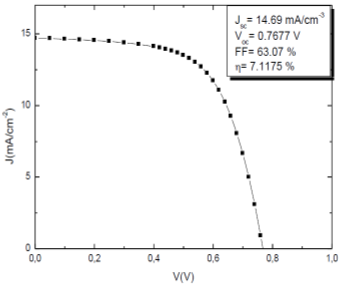Figure 2. JV characteristic of the simulated a-Si:H solar cell structure

A comparison between the experimental [13, 14] and the simulated cell performances at $\mathrm{T}=300 \mathrm{~K}$ is summarized in deduce the open-circuit voltage, $\operatorname{VOC}=0.76 \mathrm{~V}$, the current density of short circuit, JSC $=14.69 \mathrm{~mA} \mathrm{~cm}-2$, the fill factor, $\mathrm{FF}=63.07 \%$, and the efficiency, $\eta=7.11 \%$.

Table 1. Parameters used to simulate the a-Si:H solar cell

## biao_1.png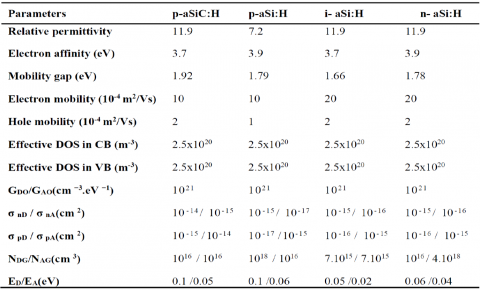Table 2. Experimental and simulated values of pin hydrogenated amorphous silicon solar cell performance [13, 14]

 Vco Jsc FF η Refs 0.80±0.02 14.10±0.43 62±2.1 7±0.28 wxAMPS-1D 0.7675 14.99 61.78 7.1098

3.3 I- layer thickness effect on the performances of the cell

Figure 3 shows the dependence of cell performances on i- layer thickness. The cell performances are: the short circuit current $\left(\mathrm{J}_{\mathrm{sc}}\right)$, the open circuit voltage $\left(\mathrm{V}_{\mathrm{oc}}\right)$, the fill factor (FF) and the conversion efficiency ($\eta$).

In reality, the maximum of open circuit voltage is limited by the recombination mechanisms. If we consider that the surfaces of the cell don't cause any phenomenon of recombination (ideal case), the main limitation of the Voc comes from the volume. Therefore, the maximum value that could be reached depends on the thickness of the cell. We deduce Voc=768mV for a cell with a i-layer thickness of 300 nm [16, 17].

The current density of short circuit increases with the intrinsic layer thickness. The widening of the intrinsic layer leads to enlargement of the photocarriers generation region as result the number of photocarriers generated by the light increases and the current density of short circuit also increases [16, 17].

Figure 4. c shows the variation of the fill factor, FF. We note a clear decrease of the FF beyond a thickness of 300nm. The FF considers the percentage of pairs collected compared to the pairs created. We can say that the a-Si: H has many defects so many trapped pairs and fewer pairs collected. Therefore, the recombination becomes more important if i-layer thickness increase. On the other hand, as an increase in this i-layer thickness implies an increase of series resistance, which decreases the fill factor.

The efficiency achieves a maximum value around 7.11% corresponding to an optimum intrinsic layer thickness about 560 nm.

3.4 The JV characteristic a-SiC:H solar cell

The conversion efficiency of a solar cell can be increased significantly with the improvement of materials properties and subsequently the designs and structures of the cell. Hydrogenated amorphous silicon carbide (a-SiC:H) alloys have been used as the top layers of the single and multijunction approaches. The ideal thin film for a window layer should have higher carrier concentration than silicon absorber, low resistively and high mobility. The a-SiC:H films have been widely investigated as a material with wide band gap.

Figure (4) shows the characteristic current-voltage curve J(V) under AM1.5 light illumination, obtained by the different optimal values of the thicknesses and concentration doping of a-SiC: H p-layer.

The conversion efficiency η of the cell presents an optimum value of 10.59% for the p-layer doping concentration.

## 3.png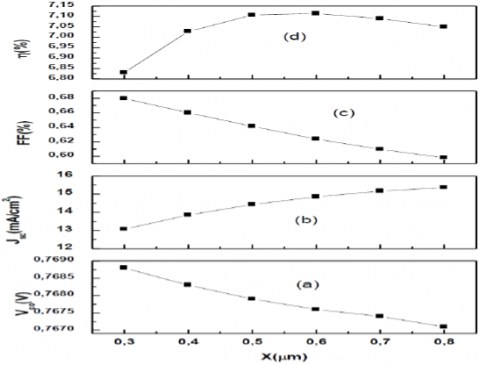Figure 3. Effect of intrinsic layer thickness on open circuit voltage (a), short-circuit current density (b), fill factor (c) and efficiency (d)

## 4.png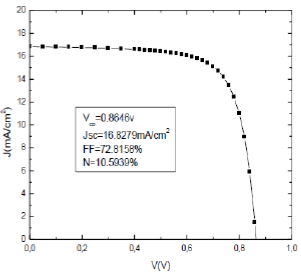Figure 4. JV characteristic

The using of wide band gap a-SiC:H as window layer is important to enhance the absorption in p-i-n solar cell-based a-Si:H, due to their high conductivity, low defect density, and wide optical band gap .

The p-layer functions as window layer through which the light enters. Photons that are absorbed in the i-layer create an electron-hole pair. The electric field induced across the i-layer by the p- and n- layers causes the electrons to drift towards the n- layer and the holes towards the p-layer. At the doped layers, the charge carriers are collected by electrical contacts and contribute to the output power of the solar cell. In the device modeling, wide bandgap a-SiC:H is used as p-doped window layer to reduce absorption losses.

Moreover, Voc also increases for its wider bandgap. It is well known that Voc is sensitive to p- layer and p/i interface. As optical absorption at the p-layer limits Jsc, wider optical gap material is always desired for improving Jsc.

Table (3) summarizes the simulated output parameters a-SiC:H(P)/a-Si:H(I)/a-Si:H(N)solar cell and compares them with the output parameters a-SiH(P)/a-Si:H(I)/a- Si:H(N)solar cell [13, 14].

Table 3. Comparison of single and heterojunction Amorphous solar cells performances

$\begin{array}{lcc} \hline \begin{array}{l} \text { Solar cell } \\ \text { performances } \end{array} & \text { Ref cell }[13,14] & \begin{array}{l} \text { Simulated } \\ \text { cell } \end{array} \\ \hline \text { Vco }(\text { V }) & 0.80 \pm 0.02 & 0.8646 \\ \text { Jsc }\left(\boldsymbol{m} \boldsymbol{A} / \mathbf{c m}^{2}\right) & 14.10 \pm 0.43 & 16.8279 \\ \text { FF }(\%) & 62 \pm 2.1 & 72.8158 \\ \text { (\%) } \boldsymbol{\eta} & 7 \pm 0.28 & 10.5939 \\ \hline \end{array}$

3.5 P-a-SiC:H layer thickness effect on the performances of the cell

It is well known that the open circuit voltage Voc and the short circuit current density Jsc strongly depend upon the thickness of a p-layer in a p-i-n a- Si solar cell. This dependency is caused by homogeneity and large optical absorption p-type a- Si:H.

Amorphous silicon carbide has a large optical band gap than a-Si:H so that the Photovoltaic performance dependence on the thickness of p-type a-SiC:H may be different from the case of a p-type a-Si:H .

To find the optimum structure, the p-layer thickness has been varied from 16 to 32 nm.

The highest efficiency of 10.12% has been obtained at a p-layer thickness of 24 nm which is shown in Figure 5.

The short-circuit current density Jsc is influenced by the thickness of p-layer as well as a-Si:H and decrease as the increase of p-layer thickness. The open circuit voltage Voc increases with the increase of p-layer thickness. For x= 24 nm, the conversion efficiency decreases mainly due to the increased optical loss and the high resistivity of the window layer with larger x. From the decreased of FF, it can be speculated that the high resistivity of the window layer with larger x reduces the photogenerated voltage by diminishing the current to the SnO2 front electrode.

The results are in good agreement with reference [ 9, 19, 20]

3.6 P-layer doping concentration effects

Figure 6 shows the characteristics of a-Si:H solar cell as a function of acceptor level in p-layer. Thickness of p-layer is fixed as 16nm, and doping concentration of p-layer varies from 1.1016cm-3 to 1.1019cm-3. Typical value of NA in the a-SiC:H p-layer is 1019cm-3. The highest conversion efficiency given by further optimization is 10.58%.

In order to collect the maximum number of electron hole pairs generated by absorbed photons, the electric field at the interface should be high, however, the recombination rate will also be high resulting a reduction of efficiency. Thus, there is trade-off between electric field and recombination rate. The increase of efficiency due to drastic increase of open circuit voltage of the device, however, it is possibly difficult to achieve higher NA than that of conventional a-SiC:H material without degradation of the film quality. The results are in good agreement with reference .

## 5.pngFigure 5. Photovoltaic characteristics for various p- layer (a-SiC:H) thickness.

## 6.png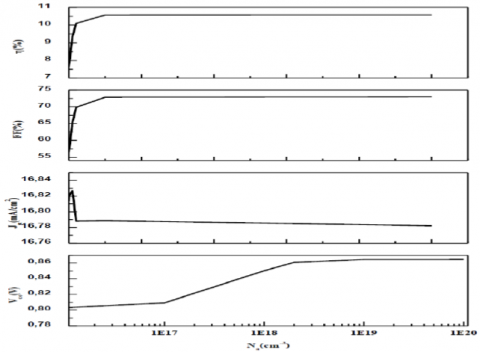Figure 6. Acceptor doping concentration in p-layer effects on photovoltaic performance

4. Conclusions

Research and development of photovoltaic solar cells is playing an ever-larger practical role in energy supply and ecological conservation all over the world. Many materials science problems are encountered in understanding exising solar cells and the development of more efficient, less costly and more stable cells.

The results of the numerical simulation were presented with a comparison between the experiment results, an acceptable agreement is obtained. The influence of the thickness of the intrinsic layer in a-Si: H solar cell on the photovoltaic parameters (Vco, Jcc, FF, η) of the solar cell has also been studied by simulation. Here, we notice that the thickness of the intrinsic layer plays a more important role than the thickness of the p- layer and n-layer in determining the final device performance.

The efficiency achieves a maximum value around 7.11% corresponding to an optimum intrinsic layer thickness about 560 nm. This makes it possible to envisage the use of amorphous layers of smaller thickness for the photovoltaic applications and it is because of absorption coefficient of a- Si: H is higher than that of the c-Si between 1.8 eV and 2,4 eV .

We have studied the effect of the a-SiC: H window layer on the performance of the photovoltaic cell based on a-Si: H. It has been found that the best structure must have a thin window layer, and a doping higher than 1019 cm-3. We have achieved the considerable initial conversion efficiency of 10.59%.

This is because the holes have a lower mobility (about 1/10 th) compared to the electrons. Since the optical generation in the i-layer is larger on the light-entry side, the holes must traverse a shorter distance to the p-layer.

Also the higher gap of a-SiC:H p-layer reduces the absorption of high energy blue wavelengths, allowing a light above blue to be absorbed into the active layer.

References

 D. Burgess, Thin-film solar cell, https://www.britannica.com/technology/thin-film-solar- cell#ref1247750, 2019.

 R. C. Chittick, J. H. Alexander, and H. F. Sterling, The Preparation and Properties of Amorphous Silicon, J. Electrochem. Soc. 116(1): 77-81, 1969.

 W. E. Spear, P. G. LeComber, S. Kinmond and M. H. Brodsky, App. Phys, Lett. 28. 105, 1976.

 Richard H. Bube, Photovoltuic material, SERIES ON PROPERTIES OF SEMICONDUCTOR MATERIALS, Vol. 1, Imperial College Press, ISBN 1 -86094-065-X, 1998.

 S. M. Iftiquar, Jeong Chul Lee, Jieun Lee, Juyeon Jang, Yeun-Jung Lee and Junsin Yi, Single- and Multiple-Junction p-i-n Type Amorphous Silicon Solar Cells with p-a-Si1-xCx:H and nc-Si:H Films, Photodiodes-From Fundamentals to Applications, doi:10.5772/51732.

 D. E. Carlson and C. R. Wronski, Amorphous silicon solar cell, Appl. Phys. Lett.; 28(11), 671 (1976).

 Deng, X., Schiff, E.A., 2003. Amorphous silicon–based solar cells. In: Luque, A., Hegedus, S. (Eds.), Handbook of Photovoltaic Science and Engineering. John Wiley & Sons.

 m. I. Kabira, zahari ibarahimb, m. Alghoulb, kamaruzzaman Sopianb, md. Rezaul karimc, nowshad amina,b,c*, bandgap optimization of absorber layers in amorphous Silicon single and multijunction junction solar cells, chalcogenide letters vol. 9, no. 1, january 2012, p. 51 – 59.

 Yoshihisa Tawada et al 1982 Jpn. J. Appl. Phys. 21 297

 Yoshihisa Tawada et al 1982 Jpn. J. Appl. Phys. 21 291

 Stephen J. Fonash, John Arch, Joe Cuiffi, Jingya Hou, William Howland, Peter McElheny, Anthony Moquin, Michael Rogosky, Thi Tran, Hong Zhu, and Francisco Rubinelli, a manual for AMPS-1D

 S. Fonash, J. Arch., J. Cuiffi, J. Hou, W. Howland, P. McElheny, A. Moquin, M. Rogosky, T. Tran, H. Zhu and F. Rubinelli, “A manual for AMPS-1D for windows 95/NT a one-dimensional device simulation program for the analysis of microelectronic and photonic structures”, The Pennsylvania State University, 1997.

 R. Martins, I. Ferreira, A. Cabrita, E. Fortunato, Improvement of a-Si:H device stability and performances by proper design of the interfaces, Journal of Non-Crystalline Solids 266±269 (2000) 1094±1098, doi: 10.1016/S0022-3093(99)00909-6.

 R. Martins, I. Ferreira, H. Aguas, V. Silva, E. Fortunato, L. Guimaraes, Engineering of a-Si:H device stability by suitable design of interfaces Solar Energy Materials & Solar Cells 73 (2002) 39–49, doi: 10.1016/S0927-0248(01)00109- X.

 Deng Qingwen, Wang Xiaoliang, Xiao Hongling, Ma Zeyu, Zhang Xiaobin, Hou Qifeng, Li Jinmin, and Wang Zhanguo, Theoretical investigation of efficiency of a p-a- SiC:H/i-a-Si:H/n-μc-Si solar cell, Journal of Semiconductors, Vol. 31, No. 10, IOPscience, doi: 10.1088/1674- 4926/31/10/103003.

 L. Ayat, S. Nour, AF. Meftah, Comparative study of a PIN homojunction a-Si:H solar cell, Journal of Ovonic Researche, Volume 15, N°1, January-February2019.

 A. Fantoni, M. Vieira, R. Martins, Simulation of hydrogenated amorphous and microcrystalline silicon optoelectronic devices,1999, doi10.1016/S0378- 4754(99)00055-5

 A. Idda, L. Ayat, N. Dahbi, Improving the performance of hydrogenated amorphous silicon solar cell using a-SiGe:H alloy, Journal of Ovonic Researche, Volume 15, N°5, January-February, 2019.

 Changwoo Lee, Harry Efstathiadis, James E. Raynolds, Pradeep Haldar, Two-dimensional Computer Modeling of Single Junction a-Si:H Solar Cells, Photovoltaic Specialists Conference (PVSC), 2009 34th IEEE, 2010, doi: 10.1109/PVSC.2009.5411215.

 Mohammed Ikbal Kabir, Seyed A. Shahahmadi, Victor Lim, Saleem Zaidi, Kamaruzzaman Sopian,and Nowshad Amin, Amorphous Silicon Single-Junction Thin-Film Solar Cell Exceeding 10%Efficiency by Design Optimization, Hindawi Publishing Corporation International Journal of Photoenergy, Volume 2012, Article ID 460919, doi:10.1155/2012/460919

 Wilfried Favre, Silicium de type n pour cellules à hétérojonctions : caractérisations et modélisations, Thèse de Doctorat, 2011,22.